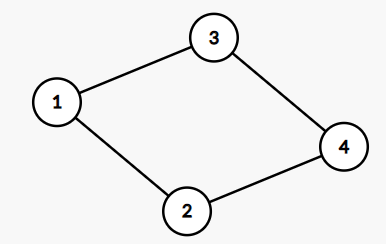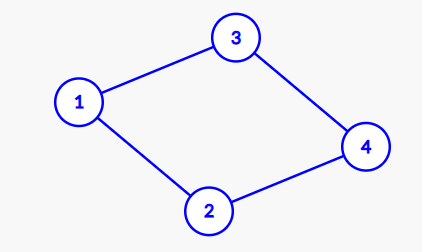# Clone of an undirected graph

## Introduction

The problem states that we need to make a clone of an undirected graph given to us.

Cloning means that all the nodes are new and don’t have nodes as references from the given original graph.

Let’s recap in brief what is an undirected graph.

A graph is a set of nodes and links between the nodes. This set of links of nodes is known as edges. If the direction of these edges is unimportant, then the graph is said to be an undirected graph.

## Sample Examples

For illustration, we show the value for graph nodes as their indexes.

Input:Output:This is a clone of an undirected graph. Although the graph looks the same, all the nodes are new and have different references.

Explanation: There are 4 nodes in the graph.

3rd node (val = 3)'s neighbors are 1st node (val = 1) and 4th node (val = 4).

4th node (val = 4)'s neighbors are 2nd node (val = 2) and 3rd node (val = 3).

1st node (val = 1)'s neighbors are 2nd node (val = 2) and 3th node (val = 3).

2nd node (val = 2)'s neighbors are 1st node (val = 1) and 4th node (val = 4

## Solution Approach(BFS + Hashmaps)

The idea is to use the graph’s Breadth-First Search(BFS). We will visit the nodes, and while visiting any node, we will make a copy of the original node for each. If a node is already visited, it simply means that a clone for that node is already present. We will use hashmaps to maintain all the nodes that have been created. We will store the reference of an original node in the key of hashmaps and a cloned node in the value of the corresponding key. After making the copy and maintaining the order, the critical task is to connect the cloned node in the same order as the original graph.

While visiting the neighbor vertices of a node ‘U,’ in the original graph, also get the corresponding cloned node for ‘U,’ let call it  V, visit all the neighbors of ‘U’, and for every neighbor, get the cloned node(if not found, then create one) and then push into the vector of the cloned graph. Therefore we have a clone of an undirected graph.

Do the breadth-first search on the original and cloned graph to verify if the graph is correct. If the order of the value is the same and references are different, the cloned graph is right.

### Algorithm

1. Make a hashmap to store the reference of original and cloned nodes
2. Make a queue to do the Breadth-first search
3. Start doing the Breadth-first search
4. We will create a clone node and store the references in the hashmaps whenever the node is not visited.
5. Finally, add these neighbors to the cloned graph node.

### Implementation in C++

``````// c++ program for finding clone of an undirected graph
#include<bits/stdc++.h>
using namespace std;
class Node
{
public:
int val;
//A neighbour vector which contains refernces to
//all the neighbours of a Graph Node
vector<Node*> neighbours;
Node(int val){
this->val = val;
}
};

// Function to find a clone of an undirected graph, and will return the reference
// to the src node of the cloned graph.
Node *cloneGraph(Node *src)
{
//A hashmap to keep track of all the
//nodes that have already been created
map<Node*, Node*>mp;
queue<Node*> q;

// push the source node
q.push(src);
Node *node = new Node(src->val);

// Put the clone node into the Map
mp[src] = node;
while (!q.empty())
{
// take the front node from the queue
// visit all the neighbours
Node *U = q.front();
q.pop();
vector<Node*>ve = U->neighbours;
int n = ve.size();
for (int i = 0; i < n; i++)
{
// checking if the node exist or not
if (!mp[ve[i]]){
// If not exists then create a new Node and
// put into the HashMap with reference
node = new Node(ve[i]->val);
mp[ve[i]] = node;
q.push(ve[i]);
}
// add these neighbours to the cloned graph node
mp[U]->neighbours.push_back(mp[ve[i]]);
}
}

// Return the address of cloned source node
return mp[src];
}

// utility function to build the graph given in the sample test case
Node *buildGraph()
{
Node *node1 = new Node(1);
Node *node2 = new Node(2);
Node *node3 = new Node(3);
Node *node4 = new Node(4);
vector<Node*>v;
v.push_back(node2);
v.push_back(node3);
node1->neighbours = v;
v.clear();
v.push_back(node1);
v.push_back(node4);
node2->neighbours = v;
v.clear();
v.push_back(node1);
v.push_back(node4);
node3->neighbours = v;
v.clear();
v.push_back(node3);
v.push_back(node2);
node4->neighbours = v;
return node1;
}

// A simple BFS traversal of a graph to
// check for proper cloning of the graph
{
map<Node*, bool> visit;
queue<Node*> q;
q.push(src);
visit[src] = true;
while (!q.empty())
{
Node *u = q.front();
cout << "Value of Node " << u->val << "\n";
cout << "Address of Node " <<u << "\n";
q.pop();
vector<Node *> v = u->neighbours;
int n = v.size();
for (int i = 0; i < n; i++)
{
if (!visit[v[i]])
{
visit[v[i]] = true;
q.push(v[i]);
}
}
}
cout << endl;
}

// Driver program to test above function
int main()
{
Node *src = buildGraph();
cout << "BFS Traversal before cloning\n";
Node *newsrc = cloneGraph(src);
cout << "BFS Traversal after cloning\n";
return 0;
}``````

Output:

``````BFS Traversal before cloning
Value of Node 1
Value of Node 2
Value of Node 3
Value of Node 4

BFS Traversal after cloning
Value of Node 1
Value of Node 2
Value of Node 3
Value of Node 4

Explanation:
The output is the same in BFS for both the graphs, but the addresses of the nodes are different, which means that the cloned graph is correct.``````

#### Complexity Analysis

Time Complexity: O( | V | + | E |)

V is the number of vertices in the graph section that needs to be cloned. E the number of adjacent edges coming off vertices. Therefore we will touch V nodes and traverse E edges. Hence complexity is O(|V| + |E|) for finding the clone of an undirected graph.

Space Complexity: O(| V | + | E |)

The cloned graph we return (if we include it in the space complexity) will be the sum of the space of the cloned vertices and edge relationships that each node must maintain.

Without the result it is O( | V | ) because we will store V vertices in the hashtable (and the queue can hold at worst some fractional multiple of the total number for vertices...imagine 1 node connected to 9 nodes all at once in a graph of size 10...and we start from that 1 node. Our queue would have 9 nodes in it at once on the first iteration).

Q1. What is the difference between directed and undirected graph ?

Ans. The directed graph contains ordered pairs of vertices while the undirected contains unordered pairs of vertices. In a directed graph, edges represent the direction Of vertices, while edges do not represent the direction of vertices.

Q2. What is the maximum number of edges in the undirected graph of Nodes N?

Ans. Each node can have an edge with every other n-1 node in an undirected graph. Therefore the total number of edges possible are n*(n-1)/2.

Q3. Which data structure is used in the BFS and DFS of the graph?

Ans. In BFS, a queue data structure is used, while in DFS stack is used.

## Key takeaways

In this article, we discussed finding the clone of an undirected graph. We use the BFS traversal and hashmaps to store the references of the nodes to be cloned. We hope you understand the problem and solution properly. Now you can do more similar questions.

If you are a beginner, interested in coding, and want to learn DSA, you can look for our guided path for DSA, which is free!